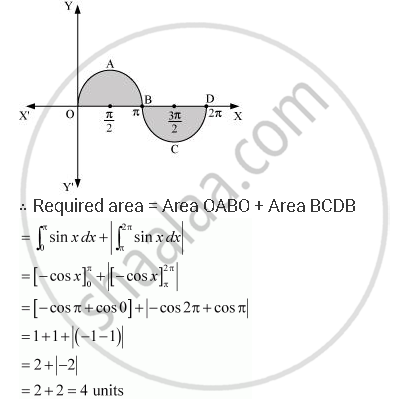Share

# Find the Area Bounded by the Curve Y = Sin X Between X = 0 and X = 2π - CBSE (Science) Class 12 - Mathematics

ConceptArea of the Region Bounded by a Curve and a Line

#### Question

Find the area bounded by the curve y = sin between x = 0 and x = 2π

#### Solution

The graph of y = sin x can be drawn asIs there an error in this question or solution?

#### APPEARS IN

NCERT Solution for Mathematics Textbook for Class 12 (2018 to Current)
Chapter 8: Application of Integrals
Q: 5 | Page no. 375

#### Video TutorialsVIEW ALL 

Solution Find the Area Bounded by the Curve Y = Sin X Between X = 0 and X = 2π Concept: Area of the Region Bounded by a Curve and a Line.
S